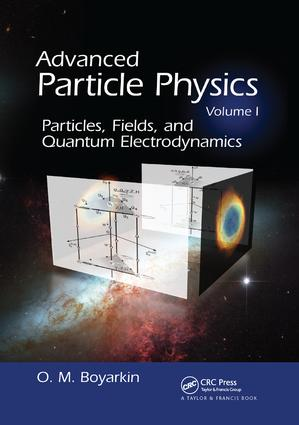# Advanced Particle Physics Volume I

## Particles, Fields, and Quantum Electrodynamics, 1st Edition

CRC Press

655 pages | 117 B/W Illus.

##### Purchasing Options:\$ = USD
Paperback: 9781138115996
pub: 2017-05-24
\$82.95
x
Hardback: 9781439804148
pub: 2011-02-16
\$220.00
x
eBook (VitalSource) : 9780429075582
pub: 2011-02-16
from \$39.98

FREE Standard Shipping!

### Description

Helping readers understand the complicated laws of nature, Advanced Particle Physics Volume I: Particles, Fields, and Quantum Electrodynamics explains the calculations, experimental procedures, and measuring methods of particle physics. It also describes modern physics devices, including accelerators, elementary particle detectors, and neutrino telescopes.

The book first introduces the mathematical basis of modern quantum field theory. It presents the most pertinent information on group theory, proves Noether’s theorem, and determines the major motion integrals connected with both space and internal symmetry. The second part on fundamental interactions and their unifications discusses the main theoretical preconditions and experiments that allow for matter structure to be established at the quark-lepton level. In the third part, the author investigates the secondary quantized theories of free fields with spin 0, 1/2, and 1, with particular emphasis on the neutrino field. The final part focuses on quantum electrodynamics, the first successfully operating quantum field theory. Along with different renormalization schemes of quantum field theory, the author covers the calculation methods for polarized and unpolarized particles, with and without inclusion of radiative corrections.

Each part in this volume contains problems to help readers master the calculation techniques and generalize the results obtained. To improve understanding of the computation procedures in quantum field theory, the majority of the calculations have been performed without dropping complex intermediate steps.

MATHEMATICAL PRELUDE

Relativistic Invariance

Three-Dimensional World

Orthogonal transformation group O(3)

Tensor representations of the SO(3)-group

The Four-Dimensional Minkowski Space

The homogeneous Lorenz group

Classification of irreducible representations of the Lorentz homogeneous group

Tensor representations of the limited homogeneous Lorentz group

Spinor representations of the homogeneous Lorentz group

Poincare group and its representations

Lagrangian Formulation of Field Theory

Principle of least action. Lagrange–Euler equations

Noether theorem and dynamic invariants

Energy-momentum tensor

Moment of momentum tensor

Electromagnetic current vector and electric charge

Isotopic spin

Discrete Symmetry Operations

Spatial inversion

Time inversion

Charge conjugation

G-parity

CPT theorem

BIRD’S-EYE VIEW ON MICROWORLD

Fundamental Interactions

Species of interactions

On path to unified field theory

Atoms — Nuclei — Nucleons

Atomism

Rutherford model of atom

Structure of atomic nucleus

From Muon to Gluon

Yukawa hypothesis

Isotopic multiplets

Unitary multiplets

Quark "Atoms"

Hypothesis of fundamental triplets

X-ray photography of nucleons

Parton model

Quantum chromodynamics. The first acquaintance

Heavy quarks and flavor symmetries

Passing Glance on Theory of Electroweak Interaction

Spontaneous symmetry breaking

Glashow–Weinberg–Salam theory

Fundamental Particles of Standard Model

Technical Equipments of Particle Physics

Accelerators

Detectors

Neutrino telescopes

QUANTUM IDYLL — FREE FIELDS

Scalar Field

Klein–Gordon equation

Quantization

Production and destruction operators

Wave-corpuscle dualism

Commutation relations. Properties of commutator functions

Microcausality

Relativistically covariant scheme of canonical quantization

Green function of scalar field

Causal Green function

Chronological product. Convolution of operators

First order equation for scalar particles

Particles with Spin 1/2

Dirac equation

Calculating γ-matrix tracks

Relativistic covariance

Solutions of free Dirac equation

Once more about particles spin

Polarization density matrix for Dirac particles

Dirac equation in external electromagnetic field

Charge conjugation, spatial inversion and time inversion for Dirac field

Relativistic quantization scheme

Dirac field quantization (momentum space)

Dirac field quantization (configuration space)

Majorana equation

Quantization of Majorana field

Second quantized representation of discrete operations C, P and T for particles with spin 1/2

Neutrino in Dirac and Majorana theory

Massive Vector Field

Proca equation

Dynamic invariants of vector field

Commutation relations in Proca theory

Duffin–Kemmer equation for vector field

Electromagnetic Field

Maxwell equations

Dynamic invariants of electromagnetic field

Electromagnetic field quantization

Quantization with use of indefinite metric

Photon polarization

QUANTUM ELECTRODYNAMICS

S-Matrix

Equations and dynamic variables in Heisenberg representation

Interaction representation

Scattering matrix

Representation of S-matrix as sum of normal products

Calculation of S-matrix elements

Feynman rules in coordinate space

Furry’s theorem

Feynman rules in momentum representation

Crossing symmetry

Cross section for unpolarized particles

Cross section for scattering by external fields

Decays of elementary particles

Cross section for polarized particles

Statistical hypothesis

Transmission of γ-Radiation through Matter

Photoeffect (nonrelativistic case)

Photoeffect (relativistic case)

Compton-effect (unpolarized case)

Compton-effect (polarized case)

Electron-positron pair production by photon in nucleus field

Resume

Scattering of Electrons and Positrons

Bremsstrahlung

Radiation of long-wave photons. Infrared catastrophe

ee+-pair annihilation into two γ-quanta. ee+-pair production under annihilation of two γ-quanta

Positronium life time

Möller scattering e+ e- → e + e

Processes e+ + e → l + l (l = e, μ, τ )

Calculations of integrals over virtual momenta

Regularization methods

Self-energy of electron

Vacuum polarization

Vertex function of the third order

Radiative corrections to scattering of electron by Coulomb nucleus field

Anomalous magnetic moment of leptons

Lamb shift

Renormalization Theory

Primitive divergent diagrams

Effective elements of Feynman diagrams

Ward identity

Extraction of divergences

QED renormalizability

Renormalization group

Consistency problems of the QED

Appendix

Problems and References appear at the end of each section.

### About the Author

Oleg Boyarkin is a professor of theoretical physics at Belarus State Pedagogical University. Dr. Boyarkin has authored several physics books and was nominated as International Scientist of the Year 2007 by the International Biographical Centre of Cambridge.

### Subject Categories

##### BISAC Subject Codes/Headings:
SCI005000
SCIENCE / Astrophysics & Space Science
SCI051000
SCIENCE / Nuclear Physics
SCI055000
SCIENCE / Physics